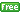www.gllamm.org

## How do I fit IRT models for polytomous responses?

 Title Fitting IRT models for polytomous responses in gllamm Author Minjeong Jeon, University of California, Berkeley Date July 2012

### IRT models for ordinal items in gllamm

Cumulative logit models, known as graded response models in item response theory, can be fitted exactly as item response models for binary responses by using the `ologit`, `oprobit`, or `ocll` (ordinal complementary log-log) links. The `thresh()` option can be used to specify different thresholds for different items. The partial credit and rating scale models use an adjacent category logit parameterization that can be implemented via the `mlogit` link (see Zheng and Rabe-Hesketh, 2007). Finally, a continuation ratio model can be fitted by expanding the data appropriately and fitting a binary logistic regression model.

#### Partial credit model (PCM)

The partial credict model is a model for polytomous items with ordered respone categories. For the PCM, we estimate step parameters for category j for item i. To fit the PCM, the data must be expanded so that each original response (j = 0, ... ,mi) is represented by mi+1 rows in the expanded dataset. Specifically, first create a new variable, `obs`, to identify each item-person combination. The data are then expanded to have one row for each response category.

```gen obs =_n
```
Suppose that there are five items and each item has 4 categories coded 0, 1, 2, and 3. Now generate variable `x` to contain all possible scores (0,1,2,3) for each item-person combination.
```expand 4
by obs, sort: generate x  = _n - 1
generate chosen = y == x
```
Then generate the variables corresponding to the design matrix for the PCM as
```forvalues i=1/5 {
forvalues g=1/3 {
gen d`i'_`g' = -1*item`i'*(x<=`g')
}
}
```
Now we are ready to fit the PCM. The syntax for the PCM can be written as
```eq slope: x
gllamm x d1_1-d5_3, i(pid) eqs(slope) link(mlogit) expand(obs chosen o) ///
```
In the first line, `eq` defines an equation corresponding to the columns of the design matrix. This equation is specified using the `eqs()` option. The `expand()` option is used to tell the program that the data have been expanded to one row for each possible response category. The variable `obs` indicates which linear preditors need to be combined for the denominator of the PCM, and the dichotomous variable `chosen` picks out the linear predictor that goes into the numerator. The multinomial logit link is specified in the `mlogit` option.

In the output, the coefficient of `d`i_j is the estimated step difficulty for item i and category j. For the two-parameter logistic (2PL) PCM, you need a different design matrix:

```forvalues i=1/5 {
gen x_it`i' = x*item`i'
}
```
The syntax to fit the 2PL PCM is
```eq load: x_it1-x_it5
```

#### Rating scale model (RSM)

The rating scale model is a special case of the PCM. The RSM assumes the differences in the step difficulties for different categories are the same for all items. The design matrix for the RSM has fewer columns than the one for the PCM. Use the same example as for the PCM, consider five items with four categories (from 0 to 3). We first need to generate the columns of the matrix that correspond to the common step parameters.

```generate step1 = -1*(x>=1)
generate step2 = -1*(x>=2)
generate step3 = -1*(x>=3)
```
The columns for the item scale parameters are generated as
```foreach var of varlist item* {
generate n`var' = -1*`var'*x
}
```
The syntax to fit the RSM is
```eq slope: x
```
The 2PL RSM has the same design matrix as the 2PL PCM. The syntax for the 2pl RCM is
```eq slope: x_it1-x_it5
```
In the RSM output, the coefficient of `nit`i is the estimated step parameter for the first step of item i. The coefficient for `step`j is the estimated additional difficulty for the step from j-1 to j where the step parameter for the first category is constrained to 0 for all items.

### Examples

• Cumulative probability models: Graded response models
• Section 8.4 on Item response models with explanatory variable in Rabe-Hesketh, S., Skrondal, A. and Pickles, A. (2004). GLLAMM Manual.U.C. Berkeley Division of Biostatistics Working Paper Series. Working Paper 160.
• Sections 11.9-11.12 on Do experts differ in their essay grading? in Rabe-Hesketh, S. and Skrondal, A. (2012). Multilevel and Longitudinal Modeling Using Stata (Third Edition). Volume II: Categorical Responses, Counts, and Survival. College Station, TX: Stata Press.
• Adjacent-category logit models: Partial credit and rating scale models

### References

• Embretson, S. E. and Reise, S. P. (2000). Item Response Theory for Psychologists. Mahwah, NJ: Lawrence Erlbaum Associates.
• Rabe-Hesketh, S. and Skrondal, A. (forthcoming). GLLAMM software. In van der Linden, W. J. and Hambleton, R. K. Handbook of Item Response Theory: Models, Statistical Tools, and Applications. Boca Raton, FL: Chapman & Hall/CRC Press, volume 3, chapter 30.
• Zheng, X. and Rabe-Hesketh, S. (2007). Estimating parameters of dichotomous and ordinal item response models using gllamm.The Stata Journal 7, 313-333.